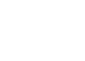﻿ ` Factorial Notation

#Factorial Notation

Recall from the previous section (Choosing Things: Does Order Matter?) that $\,{}_nC_k\,$ denotes the number of ways to choose $\,k\,$ items from $\,n\,$ (without replacement) when the order doesn't matter; it is the number of combinations that are possible when choosing $\,k\,$ items from $\,n\,$ items.

Also, $\,{}_nP_k\,$ denotes the number of ways to choose $\,k\,$ items from $\,n\,$ (without replacement) when the order does matter; it is the number of permutations that are possible when arranging $\,k\,$ items that are chosen from $\,n\,$ items.

You will see in the next section that the formulas for $\,{}_nC_k\,$ and $\,{}_nP_k\,$ involve patterns like this: $$\cssId{s7}{10 \times 9 \times 8 \times 7 \times 6 \times 5 \times 4 \times 3 \times 2 \times 1}$$

In this pattern, you start with a whole number, multiply by one less than this number, and continue until you get down to $\,1\,.$

It is tedious and inefficient to write down this pattern, particularly when the starting number is large:

30 · 29 · 28 · 27 · 26 · 25 · 24 · 23 · 22 · 21 · 20 · 19 · 18 · 17 · 16 · 15 · 14 · 13 · 12 · 11 · 10 · 9 · 8 · 7 · 6 · 5 · 4 · 3 · 2 · 1

Fortunately, there is a compact notation for this pattern, called factorial notation. We can write:

$$\cssId{s15}{10!} \cssId{s16}{= 10 \times 9 \times 8 \times 7 \times 6 \times 5 \times 4 \times 3 \times 2 \times 1}$$

The expression $\,10!\,$ is read aloud as ten factorial.

Remember that multiplication can be denoted in several ways. In the examples below, the preferred (simplest) way is shown first:

$$\cssId{s20}{3\cdot 2} \cssId{s21}{= 3\times 2} \cssId{s22}{= (3)(2)}$$ $$\cssId{s23}{ab} \cssId{s24}{= a\cdot b} \cssId{s25}{=a \times b} \cssId{s26}{=(a)(b)}$$

In the definition below, the symbol $\,:=\,$ (an equal sign, with colon in front of it) emphasizes equal, by definition.

DEFINITION factorial notation

Let $\,n\,$ be a whole number; i.e., a member of the set $\,\{0,1,2,3,\ldots\}\,.$

For $\,n\gt 1\,$: $$\cssId{s32}{n! \ :=\ n(n-1)(n-2) \times \cdots \times 1}$$ Also: $$\cssId{s34}{ \begin{gather} 1!\ :=\ 1 \cr 0!\ :=\ 1 \end{gather}}$$ The symbol ‘$\ n!\$’ is read aloud as ‘$\,n\,$ factorial’.

Another pattern that frequently arises in counting arguments is something like this: $$\cssId{s37}{30 \times 29 \times 28 \times 27 \times 26 \times 25 \times 24}$$ This pattern starts out the same as the pattern for $\,30!\,$ ($\,30\,$ factorial), but you don't continue all the way down to the number $\,1\,.$ Clearly, this pattern can also be tedious and inefficient.

Is there a way to use factorial notation to get a simpler name for this kind of pattern? Yes! The key is to multiply by $\,1\,$ in an appropriate form, as shown below:$30\cdot 29\cdot 28\cdot 27\cdot 26 \cdot 25\cdot 24$ $\displaystyle \ = 30\cdot 29 \cdot 28\cdot 27\cdot 26\cdot 25\cdot 24 \cdot \frac{23!}{23!}$ multiply by $\,1\,$ in an appropriate form $\displaystyle \ = \frac{30\cdot 29\cdot 28\cdot 27 \cdot 26\cdot 25\cdot 24\cdot 23!}{23!}$ move numbers into the numerator $\displaystyle \ = \frac{30!}{23!}$ now, we have $\,30\,$ down to $\,1\,$ in the numerator

This web exercise will give you practice with factorial notation.